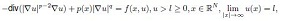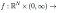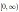##### Formulation of a Connection for Prolongations and an Application to the Burgers-KdV Equation

Paul Bracken

Journal of Advances in Mathematics and Computer Science, Page 52-61
DOI: 10.9734/BJMCS/2012/1141

The Wahlquist-Estabrook approach which has been applied to investigate the prolongation structure of many nonlinear systems is introduced. The theory which results is applied to the Burgers-KdV equation which is shown to have a nontrivial prolongation algebra. It is shown that the resulting equations can be solved to produce a very general solution. Based on the results determined for the algebra, without picking a specific representation for the algebra, a Lax pair for the equation is determined in terms of the basic generators of the algebra.

##### An Exploratory Study of K-Means and Expectation Maximization Algorithms

Journal of Advances in Mathematics and Computer Science, Page 62-71
DOI: 10.9734/BJMCS/2012/1036

In this paper, K-Means and Expectation-Maximization algorithms are part of the commonly employed methods in clustering of data in relational databases. Experiments conducted with both clustering algorithms revealed that both algorithms have been found to be characterized with shortcomings. The parameters considered in evaluating the results of findings are the number of iterations (no distinct convergence, 1), the computation time (not defined, 3.2s) and the memory space (not defined, 1.1MB) consumed at the point of convergence of both K-means and Expectation-Maximization algorithms respectively. The results obtained revealed that Expectation-Maximization algorithm’s quick and premature convergence cannot be said to have guaranteed optimality of results while K-means was found not to guarantee convergence. Though reasonable conclusion could be drawn from results obtained with Expectation-Maximization algorithm, its premature convergence may raise some questions of doubt with regards to reliability of results obtained.

##### Local Convexity Shape-Preserving Surface Data Visualization by Spline Function

Muhammad Abbas, Ahmad Abd Majid, Mohd Nain Hj Awang, Jamaludin Md Ali

Journal of Advances in Mathematics and Computer Science, Page 72-93
DOI: 10.9734/BJMCS/2012/1181

The main purpose of this paper is the visualization of convex surface data to present a smooth, visually pleasing and interactive convexity preserving surfaces. The rational cubic function with three free parameters is extended to rational bi-cubic partially blended function to preserve the shape of convex surface data. The function involves twelve free parameters in each rectangular patch. Data dependent constraints are derived for four of these parameters to preserve the shape of convex surface data while other eight are left free to user for the refinement of convexity preserving surface of data. Moreover, the scheme under discussion is , flexible, simple, local and economical as compared to existing schemes

##### Multiperiodicity Evoked by Periodic External Inputs in Cohen-Grossberg-type BAM Networks with Discrete and Distributed Delays

Yanyan Wang, Jinde Cao

Journal of Advances in Mathematics and Computer Science, Page 94-113
DOI: 10.9734/BJMCS/2012/1138

In this paper, by using contraction mapping theorem, analysis approach and decomposition of solution space, the multiperiodicity issue is discussed for Cohen-Grossberg-type (CG-type) bidirectional associative memory networks (BAMNs) with discrete and distributed delays and a general class of activation functions, where the general class of activation functions consist of nondecreasing functions with saturations including piecewise linear functions with two corner points and standard activation functions as their special cases. It is shown that for any saturation region, if there is a periodic orbit located in it, it must be locally exponentially stable. Then, based on this result, some conditions are derived for ascertaining the (n + m)-neuron CG-type BAMNs can have 2 locally exponentially stable limit cycles located in two saturation regions respectively which are symmetrical. Also, taking account of different saturation regions, results about 2(p + q) (p ≤ m, q ≤ n-1), 2min{n,m} locally exponentially stable limit cycles can be obtained, where n is the number of the neurons in one layer, m is the number of the neurons in the other layer. Meanwhile, for every locally exponentially stable limit cycle given, the corresponding saturation region can be expressed concretely. Finally, three examples are given to illustrate the effectiveness of the obtained results.

##### Ground State Solutions for A Quasilinear Elliptic Problem with a Convection Term

Qin Li, Zuodong Yang

Journal of Advances in Mathematics and Computer Science, Page 114-125
DOI: 10.9734/BJMCS/2012/1133

By a sub-supersolution argument and a perturbed argument, we improve the earlier results
concerning the existence of ground state solutions to a quasilinear elliptic problemwherefor some α ∈ (0, 1) is non-negative andis a locally Holder continuous function which may be singular at zero.